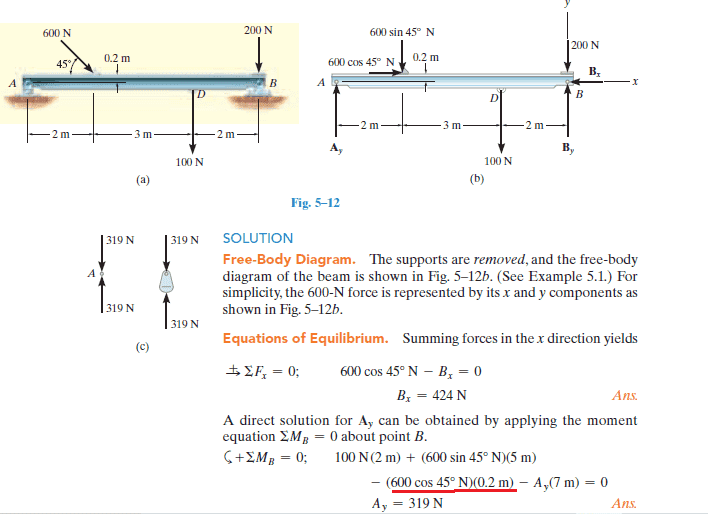# Why was the thickness of the beam included in calculating this moment?

• Engineering
• user12323567

#### user12323567

Homework Statement
Determine the horizontal and vertical components of reaction on the
beam caused by the pin at B and the rocker at A as shown in Fig. 5–12a.
Neglect the weight of the beam.
Relevant Equations
M = Fd
Hi all,

Why was the thickness of the beam considered when moment was being calculated and why was the horizontal component of the 600 N force used in calculating the moment? That part is unclear to me. Please explain it. Am I looking for the logic behind using the horizontal comp. and the thickness value for moment. Are we saying that a force applied at an angle causes some change in thickness of the beam? Please see attached picture belowBecause the point of application of the diagonal force has a vertical lever (0.2 m) that should be considered.
For the horizontal component to truly be aligned with pivot A or B, the line of application of the force should be extended until crossing the A-B line.
If that happens, the lever of the vertical component would increase to be greater than 2 meters.

Last edited: